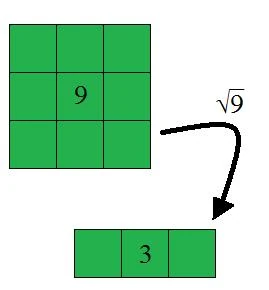## FANDOM

37 PagesThink of looking at square roots as finding the number of columns or rows a square has.

Square roots are the numbers that have been turned into squares. They are the opposite of exponents.

Square roots are usually found using the radical sign, which is $\sqrt{}\,$, though it can be found using the exponent $n^\frac{1}{2}\,$.

## Perfect SquaresEdit

Below is a list of the first ten perfect squares whose answers are whole numbers.

• $\sqrt{1}\, = 1$
• $\sqrt{4}\, = 2$
• $\sqrt{9}\, = 3$
• $\sqrt{16}\, = 4$
• $\sqrt{25}\, = 5$
• $\sqrt{36}\, = 6$
• $\sqrt{49}\, = 7$
• $\sqrt{64}\, = 8$
• $\sqrt{81}\, = 9$
• $\sqrt{100}\, = 10$

## Irrational SquaresEdit

Some square roots turn out to be irrational numbers.

• $\sqrt{2}\, = 1.41421356237309...$
• $\sqrt{3}\, = 1.73205080756887...$
• $\sqrt{5}\, = 2.23606797749979...$
• $\sqrt{6}\, = 2.449489742783178...$
• $\sqrt{7}\, = 2.645751311064591...$

## Imaginary SquaresEdit

Some square roots turn out to be imaginary numbers.

• $\sqrt{-9}\, = 3i$
• $\sqrt{-5}\, = i\sqrt{5}\,$

The square roots of negative numbers are always imaginary numbers; they have no real answer. The only way to obtain real answers from negative numbers are with cubic roots.

## See AlsoEdit

Community content is available under CC-BY-SA unless otherwise noted.